### Playing with Numbers - Solutions 1

CBSE Class –VIII Mathematics
NCERT Solutions
CHAPTER - 16
Playing with Numbers
(Ex. 16.1)

Find the values of the letters in each of the following and give reasons for the steps involved.
1.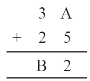Ans. On putting A = 1, 2, 3, 4, 5, 6, 7 and so on and we get, 7 + 5 = 12 in which ones place is 2.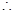A = 7
And putting 2 and carry over 1, we get
B = 6
Hence A = 7 and B = 6

2.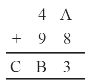Ans. On putting A = 1, 2, 3, 4, 5, 6, 7 and so on and we get,8 + 5 = 13 in which ones place is 3.A = 5
And putting 3 and carry over 1, we get
B = 4 and C = 1
Hence A = 5, B = 4 and C = 1

3.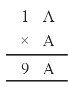Ans.  On putting A = 1, 2, 3, 4, 5, 6, 7 and so on and we get, A x A = 6 x 6 = 36 in which ones place is 6.A = 6
Hence A = 6

4.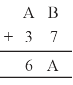Ans. Here, we observe that B = 5
so that 7 + 5 = 12.
Putting 2 at ones place and carry over 1 and A = 2, we get
2 + 3 + 1 = 6
Hence A = 2 and B = 5

5.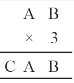Ans. Here on putting B = 0,
we get 0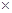3 = 0.
And A = 5, then 53 = 15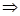A = 5 and C = 1
Hence A = 5, B = 0 and C = 1

6.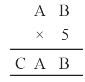Ans. On putting B = 0, we get 0 , and A = 5, then 55 = 25A = 5, C = 2
Hence A = 5, B = 0 and C = 2

7.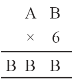Ans.  Here product of B and 6 must be same as ones place digit as B.1 = 6, 62 = 12, 63 = 18,4 = 24
On putting B = 4, we get the ones digit 4 and remaining two B’s value should be 44.For 67 = 42  and carry over 2 = 44
Hence A = 7 and B = 4

8.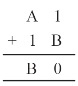Ans. On putting B = 9, we get 9 + 1 = 10
Putting 0 at ones place and carry over 1, we get
For A = 77 + 1 + 1 = 9
Hence A = 7 and B = 9

9.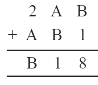Ans. On putting B = 7,7 + 1 = 8
Now A = 4, then 4 + 7 = 11
Putting 1 at tens place and carry over 1, we get
2 + 4 + 1 = 7
Hence A = 4 and B = 7

10.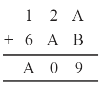Ans. Putting A = 8 and B = 1, we get
8 + 1 = 9
Now again we add 2 + 8 = 10
Tens place digit is ‘0’ and carry over 1.
Now 1 + 6 + 1 = 8 = A
Hence A = 8 and B = 1